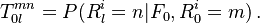# Rating Migration Matrix

## Definition

A Rating Migration Matrix (also Credit Migration Matrix, Transition Matrix) is a fundamental mathematical object used in the connection of a Credit Rating System that employs a Rating Scale. The matrix captures the probability that a certain obligor will transition (migrate) from one credit state to another over a given time period.

## Usage

Migration matrices are used in two major and distinct ways in the field of Credit Risk Modelling:

• As a purely descriptive (empirical) statistic capturing the dynamic behavior of Creditworthiness over time
• As a building block of a probabilistic Risk Model, whereby the credit quality (as expressed in probability of default or credit risk premium) is assumed to follow a Markov Chain

## Notation

A Rating migration matrix is essentially a Transition Matrix. A set of rating transition matrices encapsulates the probabilities that a name will move from rating state m at time 0 to state n at time l, i.e.,$T^{mn}_{0l} = P(R^i_l=n | F_0, R^i_0=m) \, .$

### Empirical Estimation

The simplest empirical estimation of a rating migration matrix is a frequency count

### Credit Risk Models

A transition matrix can also be used as the basic representation of stochastic credit processes.